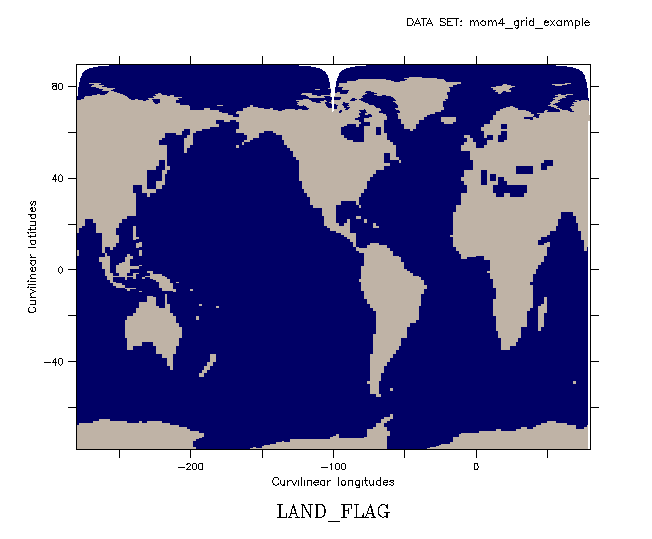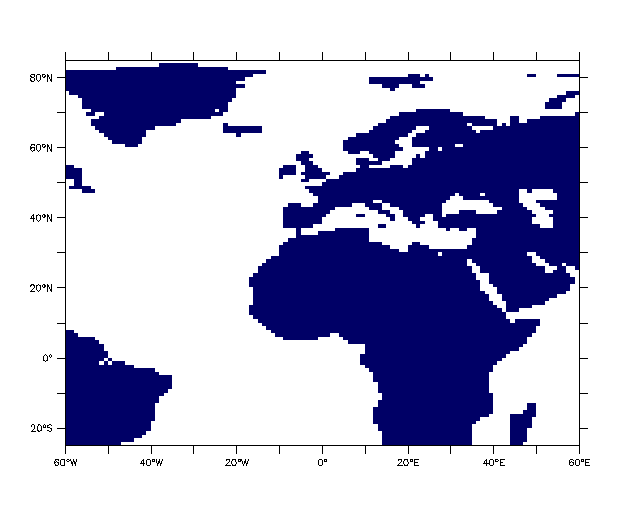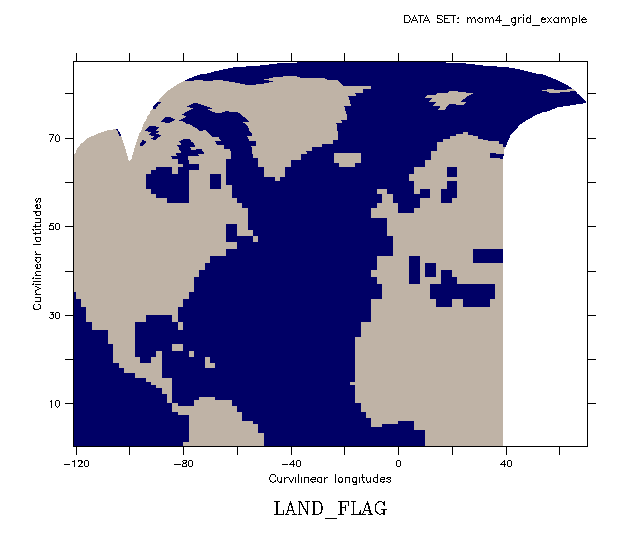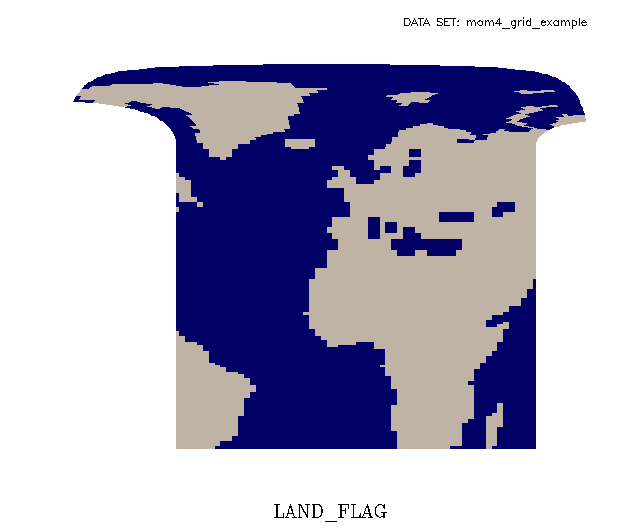# Plotting subsets of data on a curvilinear grid

## Question:

How can I determine the indices that represent longitude and latitude limits for reading a subset of data on a curvilinear grid?

## Example:

We base this example on a variable on the GFDL tri-polar grid (with some changes to the axes of the curvilinear coordinates to make them purely abstract axes). Here is a variable the on the entire grid:

```     shade/nokey/pal=land_sea land_flag, geolon, geolat```

How to specify a subset, say X=-60:60, Y=25:85 ?

If we just try SHADE/X=/Y= the plot fails, because the X= and Y= apply to the abstract X and Y axes that the curvilinear coordinates are on, and not to the data in the coordinate variables geolon and geolat.

```     shade/x=-60:60/y=25:90/nokey/pal=land_sea land_flag, geolon, geolat
**ERROR: inconsistent sizes of data regions: Y axis of X position array
```

We need instead to define a range in I and J to apply to variables land_flag, geolon, and geolat to plot a subset of the data field in curvilinear coordinates.

We want a plot corresponding to this rectilinear basemap plot:

```     go basemap x=-60:60 y=25:85 60 land_sea```

## Explanation:

The curvilinear coordinates for longitude and latitude need to be searched to find the i,j rectangle which contains our latitude, longitude region. This is not directly available from the data, as, for instance, the 2-D longitude coordinate variable contains a different range of longitudes for each j.

If we choose some rectangular region of indices, bounded by i=i1:i2, j=j1:j2, this will not in general correspond to a rectangular region in the curvilinear grid. If we arbitrarily look at a plot with some range of I and J:

```     shade/nokey/i=80:160/j=80:170/pal=land_sea land_flag, geolon, geolat```

We will wind up with some oddly-shaped plot; the goal here is to choose ranges of I and J to access a subset of the data that contains all locations in the range X=-60:60, Y=25:85. We can use the tools designed for regridding of curvilinear grids.

## Solution:

There are new functions (Ferret V5.80) to regrid data from a curvilinear to a rectangular grid. These are CURV_TO_RECT_MAP, which computes the mapping that will let us regrid from the curvilinear to the rectangular grid, and CURV_TO_RECT which applies this mapping to data fields to carry out the regridding.P>

Variable map, the result of a call to function CURV_TO_RECT_MAP, contains as part of the mapping information, the indices of the longitudes and latitudes of the curvilinear grid that correspond to the coordinates of the output grid. Variables in_curv_lon and in_curv_lat are the index values from the input curvilinear grid that correspond to the longitude and latitude of the rectangular output grid. The parameter num_neighbors is 4; the code looks around at four neighboring grid cells. (The weights are based on the distance from the source ' to the result grid points. We do not need the weights here.)

```        for each  m = 1, nlon_out
for each  n = 1, nlat_out

for each k=1, num_neighbors

MAP(m,n,k,1) = wt(m,n,k)
MAP(m,n,k,2) = in_curv_lon(m,n,k)
MAP(m,n,k,3) = in_curv_lat(m,n,k)

```
The trick we'll use is to define a rectangular grid having a 2-point output longitude axis and a 2-point latitude axis. The coordinates will be the lower and upper longitudes and latitudes of the desired region. Compute the mapping from the curvilinear grid to this rectangular grid, and then the mapping gives the index bounds we need to plot a subset of the curvilinear data.
```     ! Input data on a curvilinear grid

use "my_data/mom4_grid_example.nc"

! Define the corners of our subset: These will define
! the axes of our output grid

let xmin = -80
let xmax = 80
let ymin = -45
let ymax = 85

def axis/x=`xmin`:`xmax`/npoints=2/modulo/units=degrees xax
def axis/y=`ymin`:`ymax`/npoints=2/units=degrees yax

!  Define a variable on the output grid

let lonlatout = y[gy=yax] + x[gx=xax]

! Compute weights for the mapping

let map = curv_to_rect_map (geolon_t, geolat_t, lonlatout, 10)

! The variable map includes the indices within the source
! grid which correspond to coordinates in the destination grid.
! These are the lower lon and lat and upper lon and lat indices:

! e.g. here are the longitude indices (L=2) and latitude indices (L=3)
! the four nearest neighbors (K=1:4) in the curvilinear grid, which map
! to the i=1,j=1 location on the output axes.

LIST/I=1/J=1/L=2:3 map

1      2      3      4
2   / 2:  100.0  101.0  100.0  101.0
3   / 3:   38.0   38.0   37.0   37.0

! Compute the minimum and maximum indices in I and J needed
! to plot the entire region

let llon = map[i=1,j=@min,l=2,k=@min]
let llat = map[i=@min,j=1,l=3,k=@min]

let ulon = map[i=2,j=@max,l=2,k=@max]
let ulat = map[i=@max,j=2,l=3,k=@max]

! these indices to constrain the data, longitudes and latitudes.

geolon_t[i=`llon`:`ulon`,j=`llat`:`ulat`], \
geolat_t[i=`llon`:`ulon`,j=`llat`:`ulat`]```

Now, what if the region we request crosses the branch point of the original data? Ferret's 3-argument plot commands for curvilinear coordinates do not handle modulo operations. The curvilinear regridding does work with modulo longitudes and returns the correct indices. (CURV_TO_RECT, the function that applies the mapping to regrid data, will correctly regrid data to the output rectangular grid). Say we want to plot the data from longitudes 0 to 120. This data set has its branch point at 80E.

```     ! Redefine the limits and the output axes

let xmin = 0
let xmax = 120
let ymin = -40
let ymax = 80
def axis/x=`xmin`:`xmax`/npoints=2/modulo/units=degrees xax
def axis/y=`ymin`:`ymax`/npoints=2/units=degrees yax
```

When we compute the lower and upper longitude indices, llon and ulon, the lower index is larger than the upper index. In this example, the index corresponding to the longitude minimum of 0 degrees is 140 and the index corresponding to the longitude maximum of 120 degrees is 29. We will need to draw the plot in two steps. Create two viewports with the /axes qualifier, sized in proportion to the size of the two segments. First plot the portion from index 1 to the index corresponding to the lower longitude, then the portion from the index corresponding to the upper longitude to the uppermost index in the grid.

```     IF `llon GE ulon` THEN

say "Indices for the longitude min, max are `llon`, `ulon`"
say "Requested region crosses branch point of curvilinear data."
say "Draw the plot in two sections"
say " "
say "First section:  index i = 1 to `llon`"
say "Second section: index i = `ulon` to `geolon,r=isize`"

! First section:  index i = 1 to 141
! Second section: index i = 29 to 180
ENDIF
```
Putting together sections of curvilinear data will require defining custom viewports and is not covered in this FAQ.